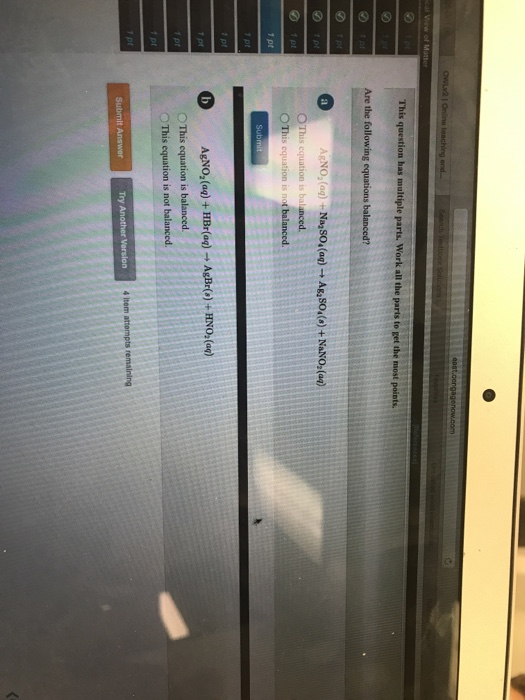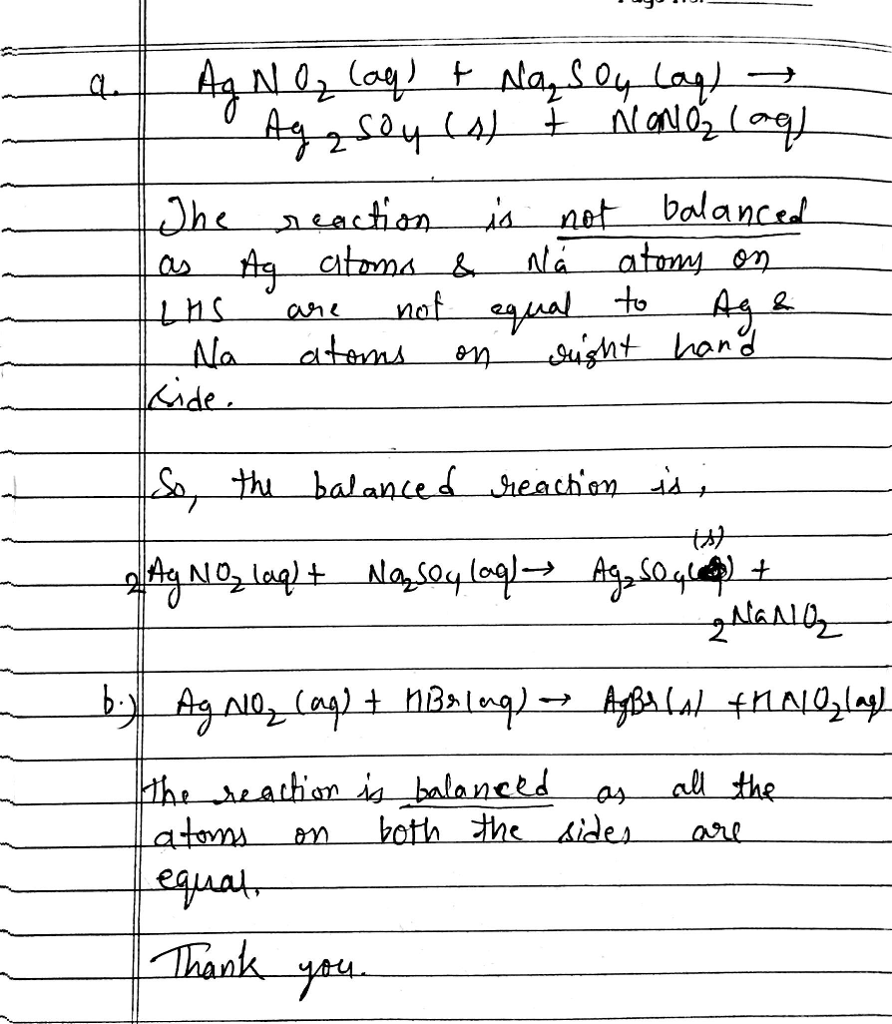# Question & Answer: Are the following equations balanced? AgNO_2(aq) + Na_2SO_4(aq) rightarrow Ag_2SO_4(s)…..Are the following equations balanced? AgNO_2(aq) + Na_2SO_4(aq) rightarrow Ag_2SO_4(s) + NaNO_2(aq) This equation is balanced. This equation is not balanced. AgNO_2(aq) + HBr(aq) rightarrow AgBr(s) + HNO_2(aq) This equation is balanced. This equation is not balanced.